﻿ 基于虚拟力引导的人机协同目标抓取方法
«上一篇文章快速检索 高级检索

 智能系统学报2021, Vol. 16Issue (4): 683-689  DOI: 10.11992/tis.2020070460

### 引用本文JIANG Tongwei, JIANG Yong. Human-machine cooperative object grasping method based on virtual force guidance[J]. CAAI Transactions on Intelligent Systems, 2021, 16(4): 683-689. DOI: 10.11992/tis.202007046.### 文章历史

1. 沈阳建筑大学 信息与控制工程学院，辽宁 沈阳 110168;
2. 中国科学院 网络化控制系统重点实验室，辽宁 沈阳 110016;
3. 中国科学院 沈阳自动化研究所，辽宁 沈阳 110016;
4. 中国科学院 机器人与智能制造创新研究院，辽宁 沈阳 110169

Human-machine cooperative object grasping method based on virtual force guidance
JIANG Tongwei 1,2,3,4, JIANG Yong 2,3,41. School of Information and Control Engineering, Shenyang Jianzhu University, Shenyang 110168, China;
2. Key Laboratory of Networked Control Systems, Chinese Academy of Sciences, Shenyang 110016, China;
3. Shenyang Institute of Automation, Chinese Academy of Sciences, Shenyang 110016, China;
4. Institutes for Robotics and Intelligent Manufacturing, Chinese Academy of Sciences, Shenyang 110169, China
Abstract: The traditional artificial potential field method in force feedback teleoperation cannot adapt to the overall obstacle avoidance of manipulators, and operators find it difficult to control manipulators to reach the required pose in the operation process. Accordingly, this paper proposes a human-machine cooperative object grasping method based on virtual force guidance. Force feedback devices provide operators with haptic interaction. By combining the artificial potential field method and virtual fixture and by constructing a pipe-like virtual force field, the real-time virtual force guidance is generated, which can assist operators in completing the overall obstacle avoidance tasks of slave robots and guide robots to return to the predefined path and approach the target point after completing their tasks. When a grasping task is performed, a virtual cone-shaped force field is constructed to help operators make manipulators reach the target position and pose. In addition, a method of limiting robot motion is proposed to reduce the impact of operators’ errors on their grasping tasks. Experimental results show that this method can effectively improve the success rate and efficiency of object grasping operation.
Key words: virtual force guidance    force feedback    motion constraints    obstacle avoidance    object grasping    master-slave control    teleoperation    human-machine collaboration

1 基于虚拟力引导目标抓取方法

1.1 管道形虚拟力场的构建

 $\left\| {P(s({{\boldsymbol{x}}_{{c}}}) - {{\boldsymbol{x}}_{{c}}})} \right\| = \min \left\| {P(s) - {{\boldsymbol{x}}_{{c}}}} \right\|$ (1)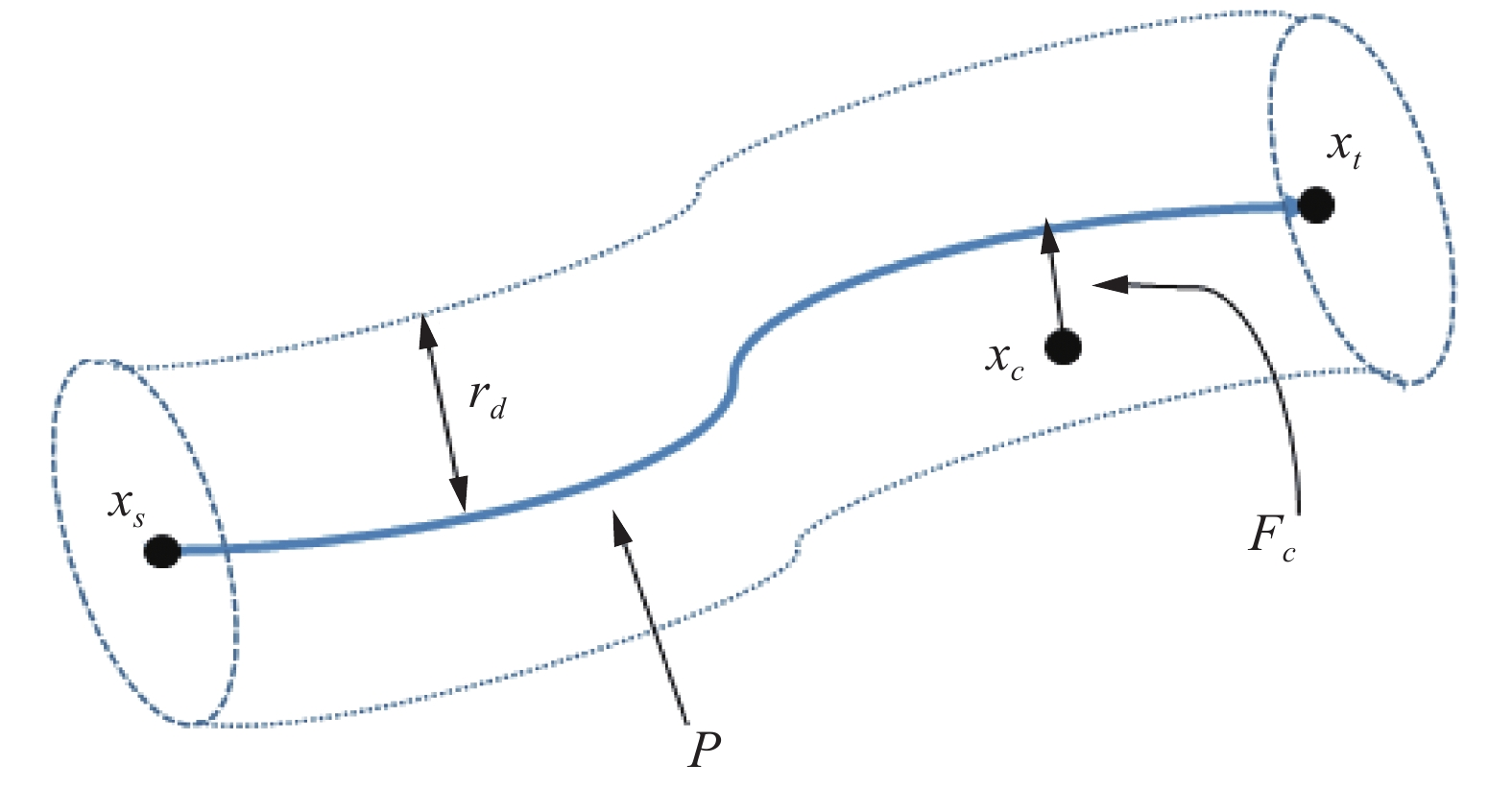Download: 图 1 管形虚拟力场示意 Fig. 1 Virtual force field of pipe

 ${F_c} = {k_c}\delta ({{\boldsymbol{x}}_{{c}}})$ (2)

 $\delta ({{\boldsymbol{x}}_{{c}}}) = {{\boldsymbol{x}}_{{c}}} - s({{\boldsymbol{x}}_{{c}}})$ (3)
1.2 避障任务力引导的构建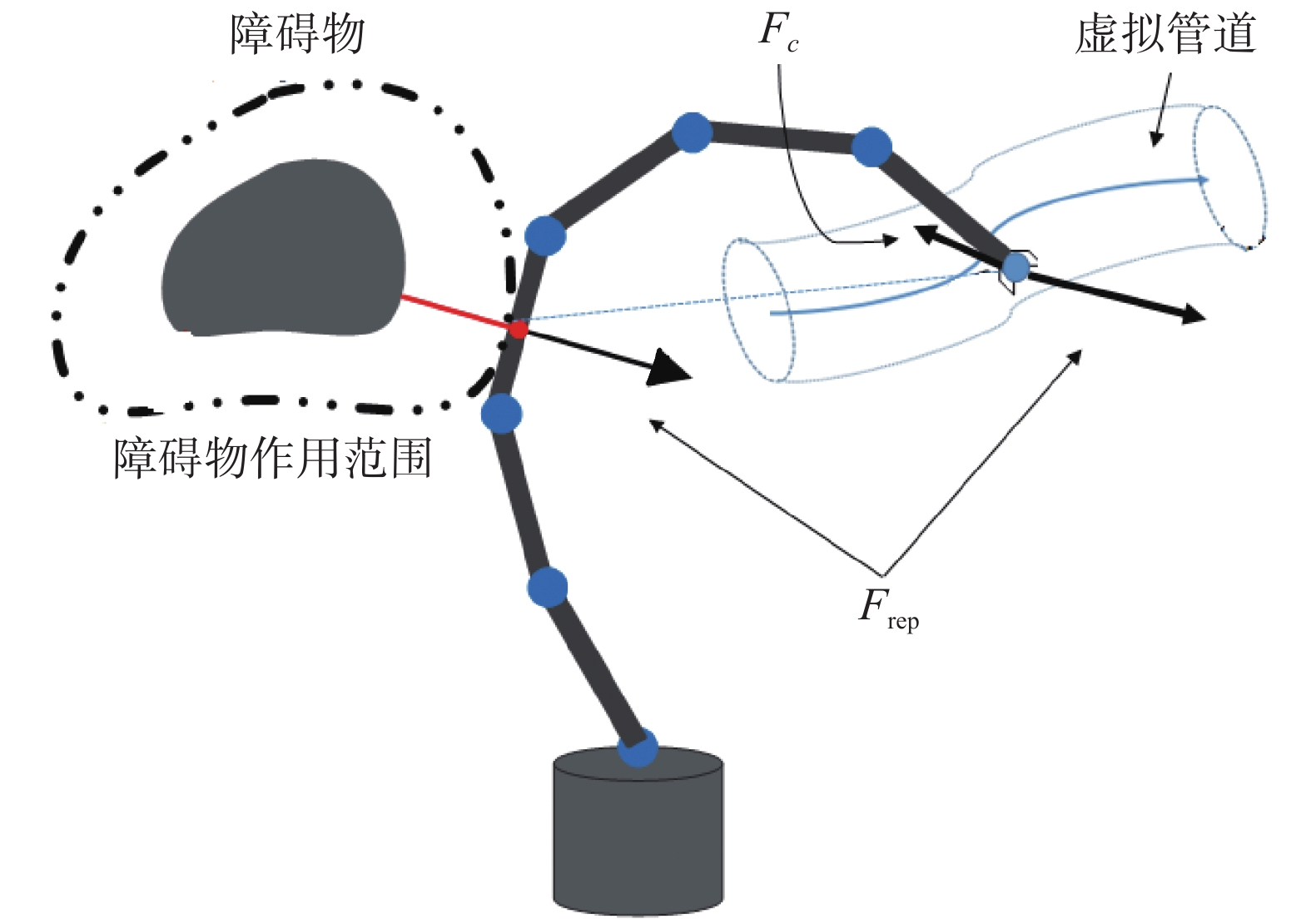Download: 图 2 避障任务的虚拟力引导 Fig. 2 Virtual force guidance for obstacle avoidance

 {{\boldsymbol{U}}_{{\rm{rep}}}} = \left\{ \begin{aligned} & {\frac{1}{2}{\eta _{{\rm{rep}}}}{{\left(\frac{1}{\rho } - \frac{1}{{{\rho _0}}}\right)}^2},}\quad{\rho < {\rho _0}} \\ & {0,}\quad{\rho \geqslant {\rho _0}} \end{aligned}\right. (4)

 {{\boldsymbol{F}}_{{\rm{rep}}}} = - \nabla {{\boldsymbol{U}}_{{\rm{rep}}}} = \left\{ \begin{aligned} & {{\eta _{{\rm{rep}}}}\left(\frac{1}{\rho } - \frac{1}{{{\rho _0}}}\right)\frac{1}{{{\rho ^2}}}\nabla \rho ,}\quad{\rho < {\rho _0}} \\ & {0,}\quad{\rho \geqslant {\rho _0}} \end{aligned} \right. (5)

 ${{\boldsymbol{F}}_{{\rm{to}}}} = {{\boldsymbol{F}}_{{c}}} + {{\boldsymbol{F}}_{{\rm{rep}}}}$ (6)

 ${{\boldsymbol{F}}_{{\rm{to}}}} = {{\boldsymbol{F}}_{{c}}} + \sum\limits_{i = 1}^n {{{\boldsymbol{F}}_{i{\rm{,rep}}}}}$ (7)

1.3 锥形虚拟力场的构建

 ${\boldsymbol{\alpha }} = \frac{{({{\boldsymbol{x}}_{{t}}} - {{\boldsymbol{x}}_{{s}}})}}{{\left\| {{{\boldsymbol{x}}_{{t}}} - {{\boldsymbol{x}}_{{s}}}} \right\|}}$ (8)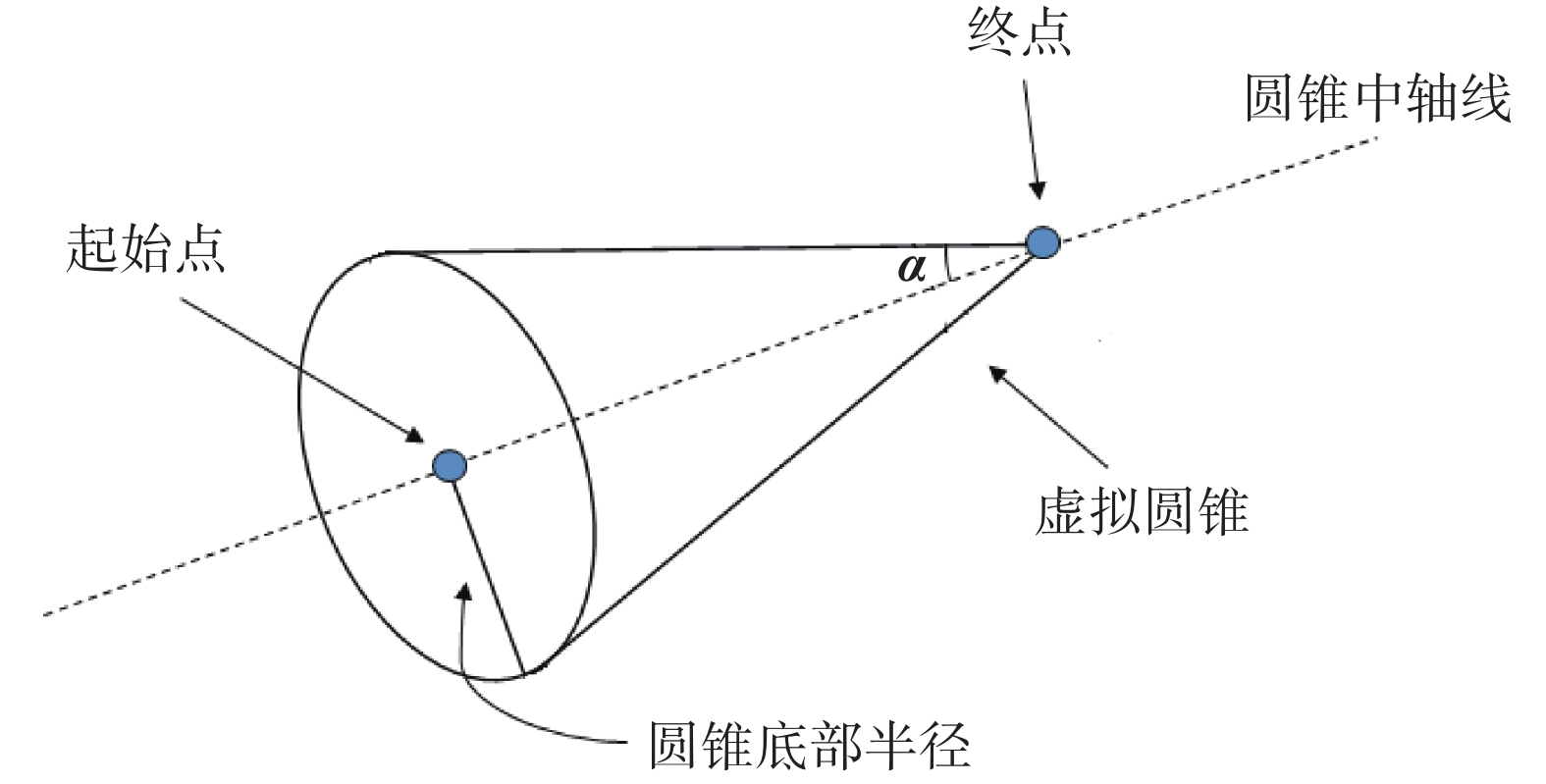Download: 图 3 锥形虚拟力场示意 Fig. 3 Conical virtual force field

 ${{\boldsymbol{n}}_{{o}}}({{\boldsymbol{x}}_{{c}}}) = ({\boldsymbol{I}} - {\boldsymbol{a}}{{\boldsymbol{a}}^{\rm{T}}})({{\boldsymbol{x}}_{{t}}} - {{\boldsymbol{x}}_{{c}}})$ (9)
 ${{\boldsymbol{n}}_{{R}}}({{\boldsymbol{x}}_{{c}}}) = ({\boldsymbol{a}}{{\boldsymbol{a}}^{\rm{T}}})({{\boldsymbol{x}}_{{t}}} - {{\boldsymbol{x}}_{{c}}})$ (10)

 ${{\rm{condition}}_{{\rm{in}}}}({{\boldsymbol{x}}_{{c}}}) \equiv \left\| {{{\boldsymbol{n}}_{{O}}}({{\boldsymbol{x}}_{{c}}})} \right\| \leqslant {d_{{\rm{cone}}}}$ (11)
 ${d_{{\rm{cone}}}} = \left\| {{{\boldsymbol{n}}_{{R}}}({{\boldsymbol{x}}_{{c}}})} \right\|\tan \alpha$ (12)

${{\boldsymbol{x}}_{{c}}}$ 在当前构建的锥形力场范围之外，即 $\left\| {{{\boldsymbol{n}}_{{O}}}({{\boldsymbol{x}}_{{c}}})} \right\| > {d_{{\rm{cone}}}}$ 时可以根据当前机械臂末端的位置重新构建锥形力场协助操作者完成操作任务。

 ${{\boldsymbol{F}}_{{p}}} = {k_n}{{\boldsymbol{n}}_{{O}}}({{\boldsymbol{x}}_{{c}}}) + {k_2}{{\boldsymbol{n}}_{{R}}}({{\boldsymbol{x}}_{{c}}})$ (13)
 ${k_n} = {k_1}\left( {1 + \frac{{d_c}{{\left\| {{{\boldsymbol{n}}_{{R}}}({{\boldsymbol{x}}_{{c}}})} \right\|}^2}}{{\left\| {{d_{{\rm{cone}}}}} \right\|}}} \right)$ (14)

 ${{\boldsymbol{F}}_{{{VG}}}} = \left[ {\begin{array}{*{20}{c}} {{{\boldsymbol{F}}_{{P}}}} \\ {{{\boldsymbol{F}}_{{R}}}} \end{array}} \right] = {k_n}\left[ {\begin{array}{*{20}{c}} {{{\boldsymbol{n}}_{{O}}}({{\boldsymbol{x}}_{{c}}})} \\ {{{\boldsymbol{\theta }}_{{t}}} - {{\boldsymbol{\theta }}_{{c}}}} \end{array}} \right] + {k_2}\left[ {\begin{array}{*{20}{c}} {{{\boldsymbol{n}}_{{R}}}({{\boldsymbol{x}}_{{c}}})} \\ 0 \end{array}} \right]$ (15)
1.4 运动约束

 ${\boldsymbol{v}}(k) = {{\boldsymbol{v}}_{{O}}}(k) + {{\boldsymbol{v}}_{{R}}}(k)$ (16)
 ${{\boldsymbol{v}}_{{O}}}(k) = ({\boldsymbol{I - a}}{{\boldsymbol{a}}^{\rm{T}}}){\boldsymbol{v}}(k)$ (17)
 ${{\boldsymbol{v}}_{{R}}}(k) = ({\boldsymbol{a}}{{\boldsymbol{a}}^{\rm{T}}}){\boldsymbol{v}}(k)$ (18)

 ${\boldsymbol{v}}(k) = \mu (k){{\boldsymbol{v}}_{{O}}}(k) + {{\boldsymbol{v}}_{{R}}}(k){\rm{ }}$ (19)

 \mu (k) = \left\{ \begin{aligned} & 1,\quad{d(k) \geqslant {d_{{\rm{cone}}}}(k)} \\ & {\frac{{0.8}d(k)}{{{d_{{\rm{cone}}}}(k)}} + 0.2},\quad{0 \leqslant d(k) \leqslant {d_{{\rm{cone}}}}(k)} \end{aligned}\right. (20)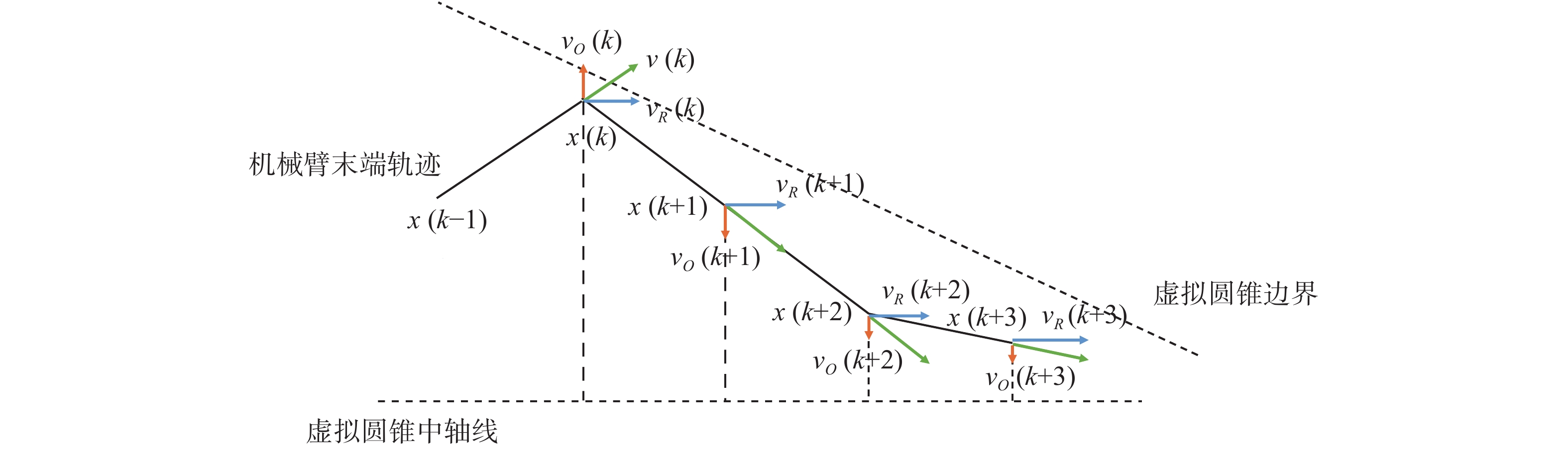Download: 图 4 速度矢量分解 Fig. 4 Examples of decomposed velocity vectors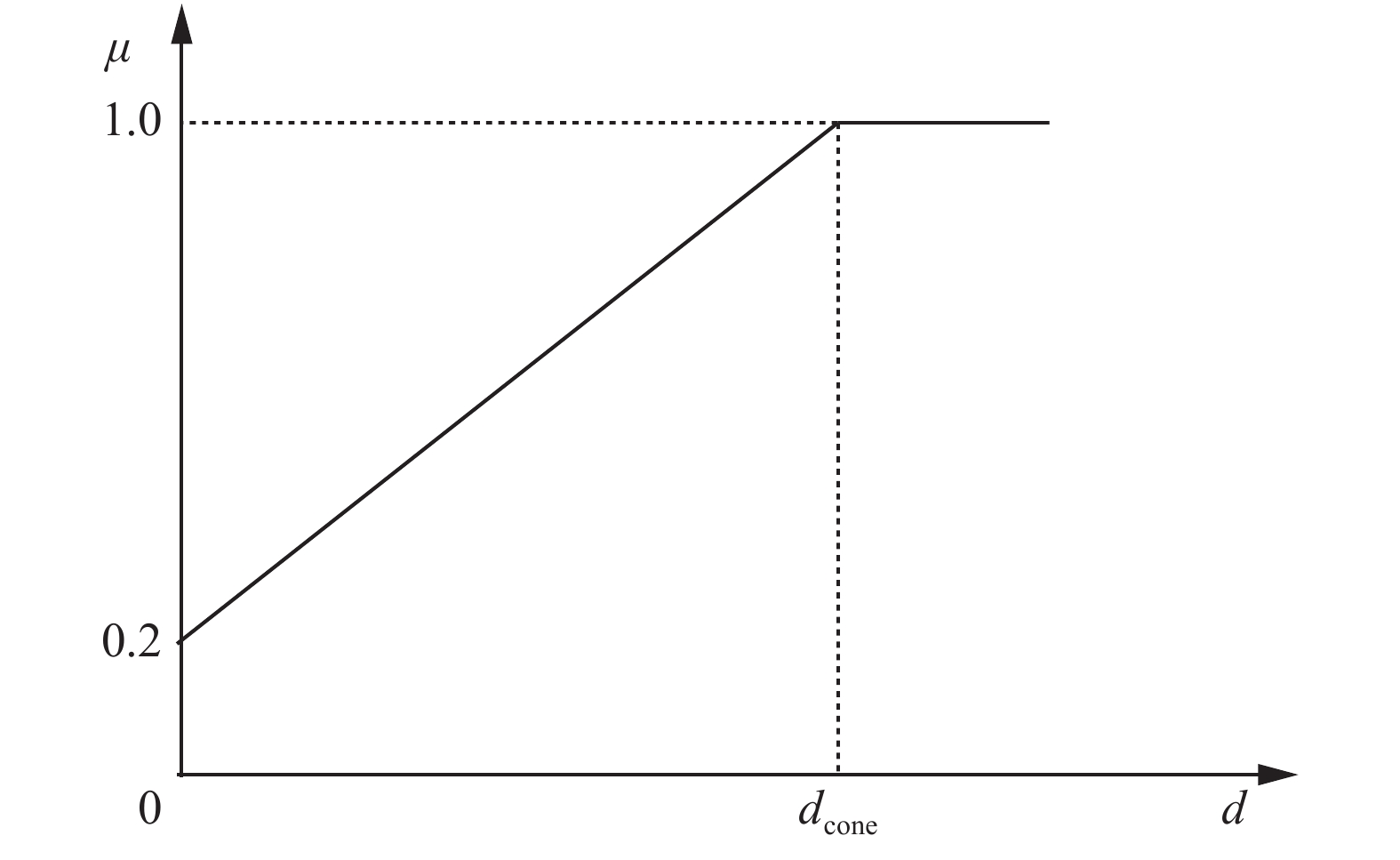Download: 图 5 参数 $\mu \left(k\right)$ 与 $d\left(k\right)$ 之间的关系 Fig. 5 Relationship between ${\mu }\left({k}\right)$ and ${d}\left({k}\right)$
2 实验验证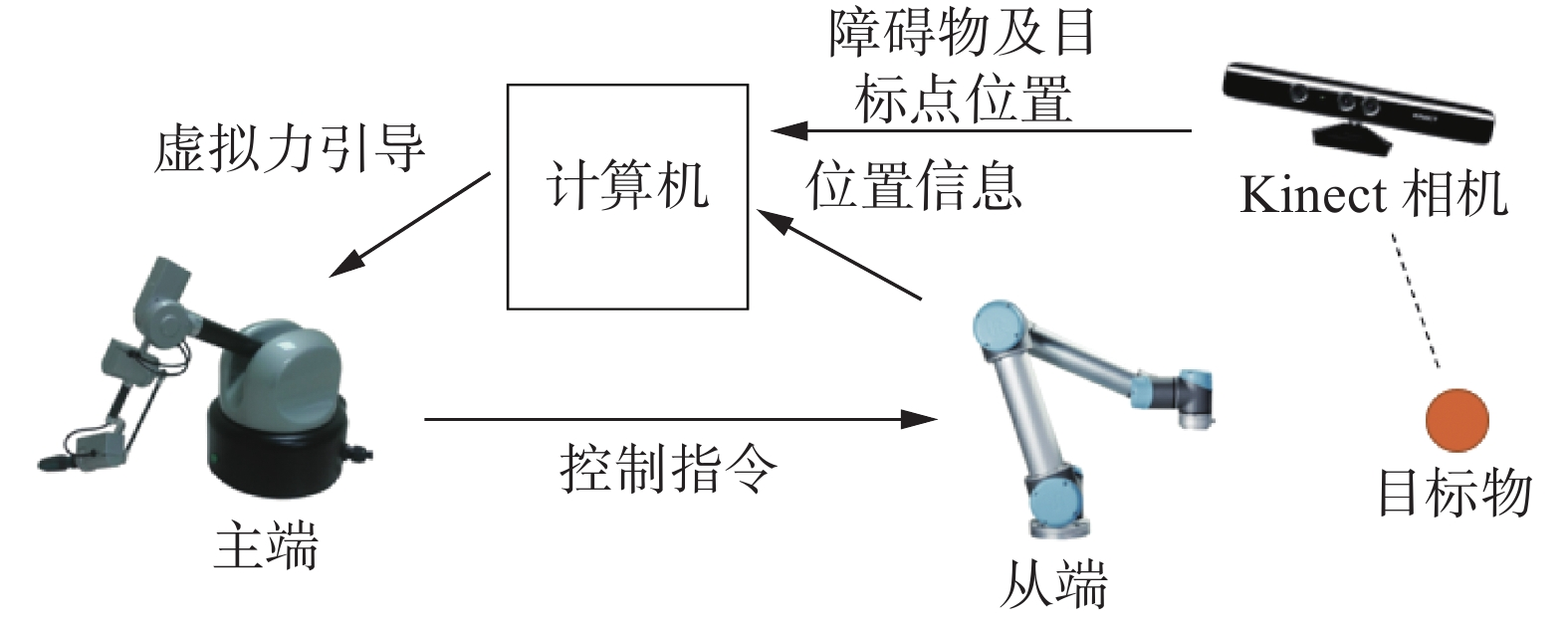Download: 图 6 虚拟引导力遥操作系统结构 Fig. 6 Virtual force guidance teleoperation system

2.1 机械臂避障实验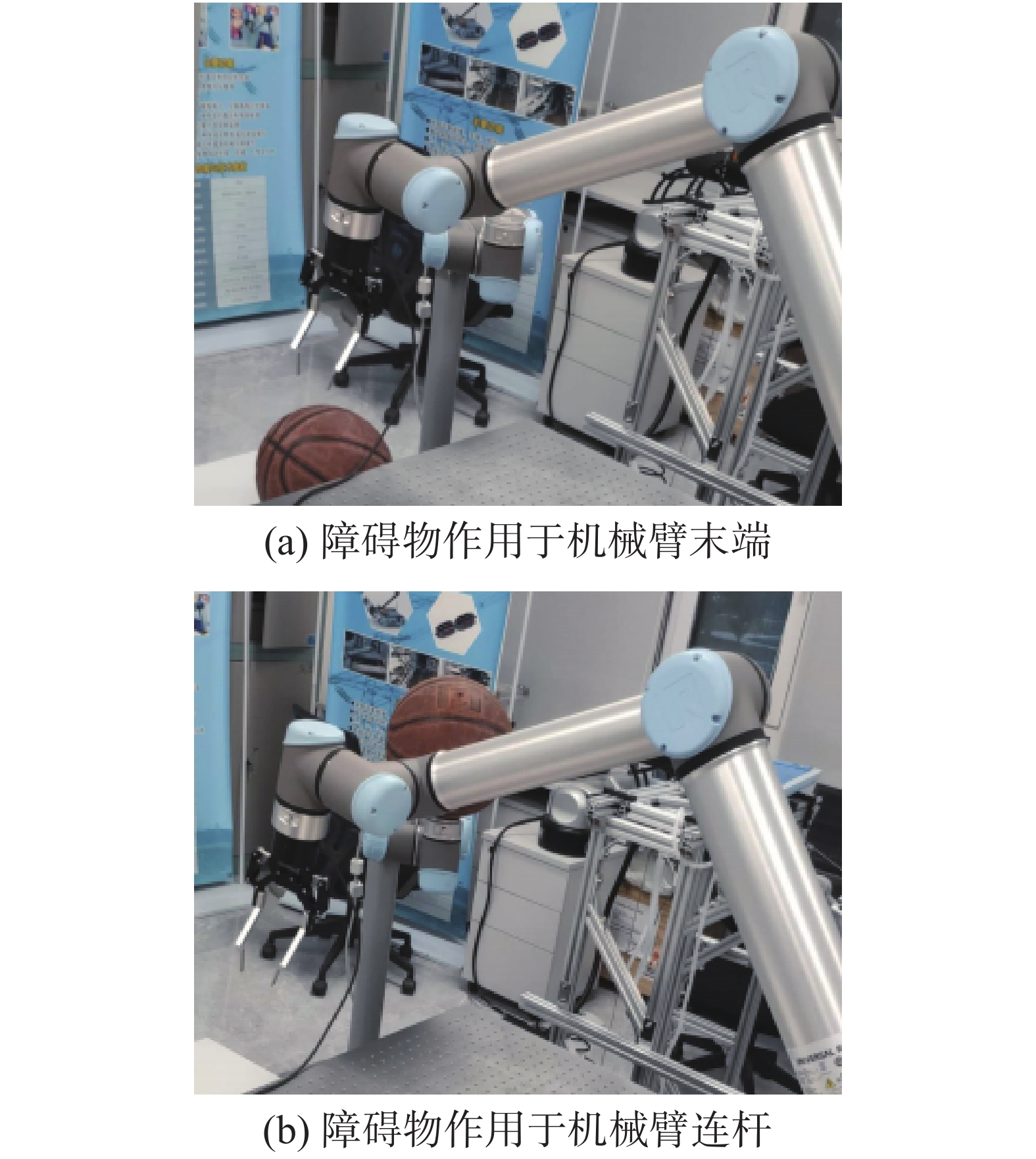Download: 图 7 机械臂避障实验的实验环境 Fig. 7 Experimental environment for obstacle avoidance表 1 障碍物作用于机械臂末端避障实验结果 Tab.1 Experimental results of the obstacle approaching the end表 2 障碍物作用于机械臂连杆避障实验结果 Tab.2 Experimental results of the obstacle approaching the linksDownload: 图 8
2.2 目标抓取验证表 3 目标抓取实验结果 Tab.3 Results of the object grasping experiment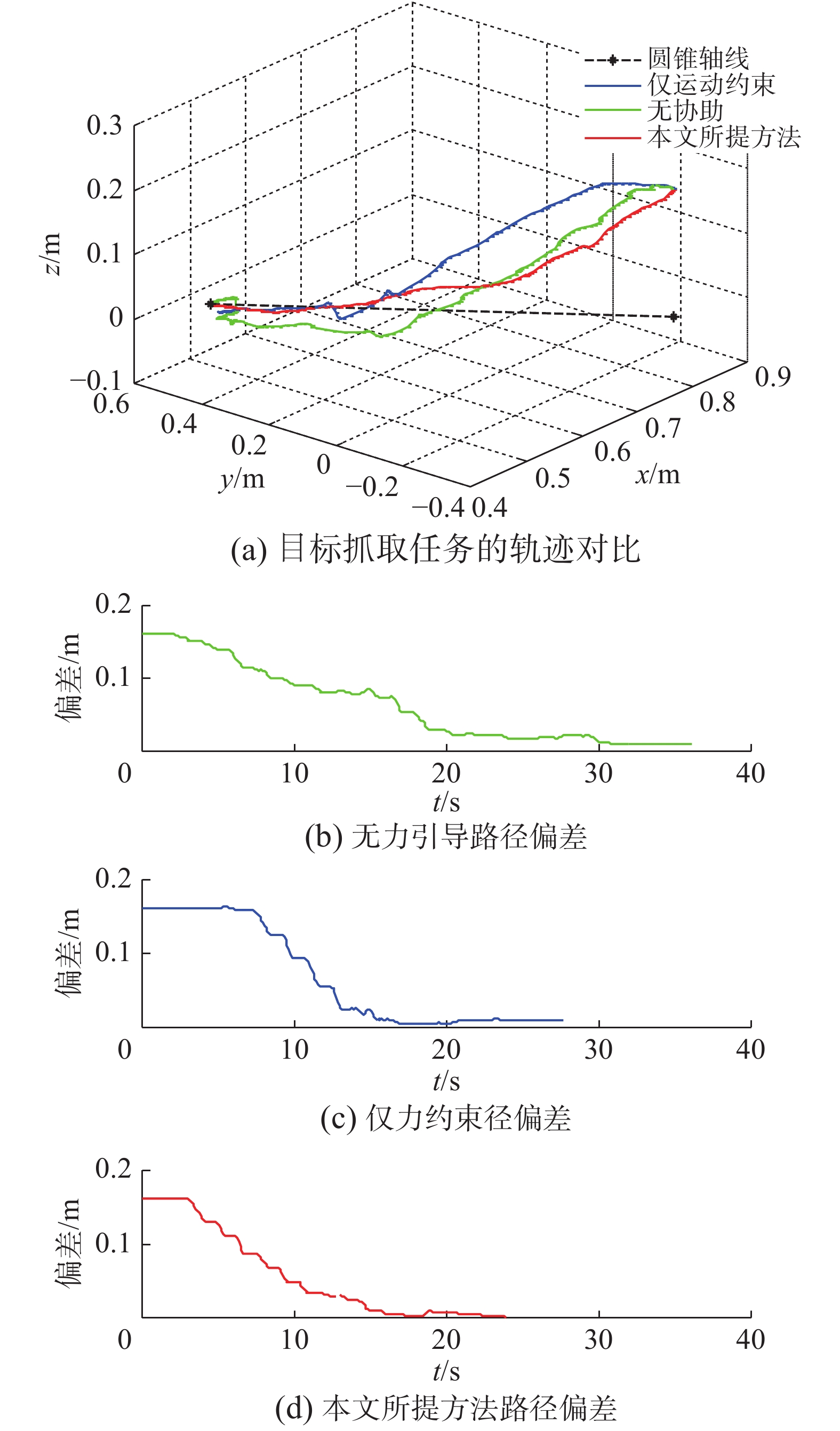Download: 图 9 不同协助下的机械臂末端轨迹 Fig. 9 Trajectory and deviation of the end of the manipulator with different assistance
3 结束语

  刘金存. 带电作业机器人主从控制的研究[D]. 济南: 山东建筑大学, 2014. LIU Jincun. Master-slave control research of live working robot[D]. Ji’nan: Shandong Jianzhu University, 2014. (0)  DRAGAN A D, SRINIVASA S S. A policy-blending formalism for shared control[J]. The international journal of robotics research, 2013, 32(7): 790-805. DOI:10.1177/0278364913490324 (0)  JAVDANI S, ADMONI H, PELLEGRINELLI S, et al. Shared Autonomy via hindsight optimization for teleoperation and teaming[J]. The international journal of robotics research, 2018, 37(7): 717-742. DOI:10.1177/0278364918776060 (0)  ROSENBERG L B. Virtual fixtures: perceptual tools for telerobotic manipulation[C]//Proceedings of 1993 IEEE Virtual Reality Annual International Symposium. Seattle, WA, USA, 1993: 76−82. (0)  BU Wanghui, LIU Guangjun, LIU Chengju. Online generation of virtual fixture for bilateral teleoperation based on intention recognition[C]//Proceedings of 2016 International Conference on Advanced Robotics and Mechatronics (ICARM). Macau, China, 2016: 122−126. (0)  LEE K H, PRUKS V, RYU J H. Development of shared autonomy and virtual guidance generation system for human interactive teleoperation[C]//Proceedings of the 14th International Conference on Ubiquitous Robots and Ambient Intelligence (URAI). Jeju, Korea (South), 2017: 457−461. (0)  ROZENBLIT J W, FENG C, RIOJAS M, et al. The computer assisted surgical trainer: design, models, and implementation[C]//Proceedings of 2014 Summer Simulation Multiconference. Monterey, USA, 2014: 211−220. (0)  BALACHANDRAN R, KOZLOVA N, OTT C, et al. Non-linear local force feedback control for haptic interfaces[J]. IFAC-PapersOnLine, 2018, 51(22): 486-492. DOI:10.1016/j.ifacol.2018.11.587 (0)  NIKOLAIDIS S, ZHU Yuxiang, HSU D, et al. Human-robot mutual adaptation in shared autonomy[C]//Proceedings of the 12th ACM/IEEE International Conference on Human-Robot Interaction (HRI). Vienna, Austria, 2017: 294−302. (0)  WANG Xinyu, YANG Chenguang, MA Hongbin, et al. Shared control for teleoperation enhanced by autonomous obstacle avoidance of robot manipulator[C]//Proceedings of 2015 IEEE/RSJ International Conference on Intelligent Robots and Systems (IROS). Hamburg, Germany, 2015: 4575−4580. (0)  HONG M, ROZENBLIT J W. A haptic guidance system for Computer-Assisted Surgical Training using virtual fixtures[C]//Proceedings of 2016 IEEE International Conference on Systems, Man, and Cybernetics (SMC). Budapest, Hungary, 2016: 2230−2235. (0)  LIU Manlu, LING Qiang, ZHANG Jing, et al, Bilateral control of teleoperation manipulator based on virtual force aware guidance[C]//Proceedings of 2017 IEEE International Conference on Cybernetics and Intelligent Systems (CIS) and IEEE Conference on Robotics, Automation and Mechatronics (RAM). Ningbo, China, 2017: 231−236. (0)  BOWYER S A, DAVIES B L, BAENA F R. Active constraints/virtual fixtures: a survey[J]. IEEE transactions on robotics, 2014, 30(1): 138-157. DOI:10.1109/TRO.2013.2283410 (0)  WANG Wenrui, ZHU Mingchao, WANG Xiaoming, et al. An improved artificial potential field method of trajectory planning and obstacle avoidance for redundant manipulators[J]. The international journal of advanced robotic systems, 2018, 15(5). DOI:10.1177/1729881418799562 (0)  BETTINI A, MARAYONG P, LANG S, et al. Vision-assisted control for manipulation using virtual fixtures[J]. IEEE transactions on robotics, 2004, 20(6): 953-966. DOI:10.1109/TRO.2004.829483 (0)  MARAYONG P, BETTINI A, OKAMURA A. Effect of virtual fixture compliance on human-machine cooperative manipulation[C]//Proceedings of 2002 IEEE/RSJ International Conference on Intelligent Robots and Systems. Lausanne, Switzerland, 2002: 1089−1095. (0)  KIM D J, HAZLETT-KNUDSEN R, CULVER-GODFREY H, et al. How autonomy impacts performance and satisfaction: results from a study with spinal cord injured subjects using an assistive robot[J]. IEEE transactions on systems, man, and cybernetics - part A: systems and humans, 2012, 42(1): 2-14. DOI:10.1109/TSMCA.2011.2159589 (0)  YOU E, HAUSER K. Assisted teleoperation strategies for aggressively controlling a robot arm with 2D input[M]//DURRANT-WHYTE H, ROY N, ABBEEL P. Robotics: Science and Systems VII. Los Angeles: MIT Press, 2012: 354−361. (0)